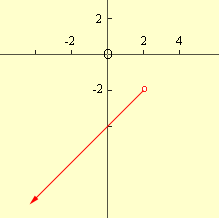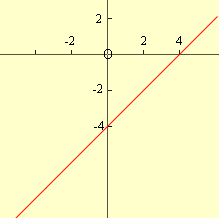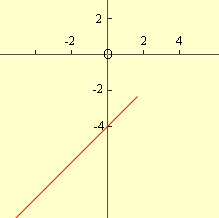Quandaries and Queries Hi, my name is Dani.  I'm in tenth grade Algebra 2, and I'm learning about a lot of different kinds of functions, and I can't quite seem to understand how every part of the piecewise function works.  I understand most of it, I think, but I've been looking at an example in my math book, and I'm not sure how they got the answer they did.  The part I don't understand reads:   "Graph f(x) = x-4 if x<2" and "Identify the domain and range."  The picture in the book shows an open circle at (2,-2), with an arrow going down and to the left with the slope being one.  How  did they get the open circle to be at (2,-2), and why does the arrow point where it does?    Thanks! Hi Dani, I think the diagram you are describing isWhen I look at this problem and the expression is y = x - 4 I see the line with slope 1 and through the point (0,-4), that is I seeBut the function described in the problem is only defined for x < 2 so I should erase the part of the line that has x-xalues larger than or equal to 2.But from this diagram alone I can't tell if the function is defined at x=2 or not. There might be a point (2,-4) on the graph, I can't tell. To show that the function is not defined at 2 one technique is to put a small open circle at the "missing point", (2,-4). Penny Go to Math Central